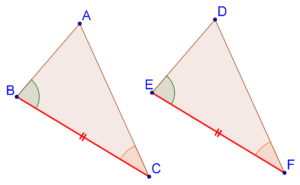# The ASA Criterion

Go back to  'Triangles'

## Introduction to Congruency:

If two triangles are congruent it means that:

• Three sides of one triangle will be (respectively) equal to the three sides of the other
• Three angles of one triangle will be (respectively) equal to the three angles of the other

However, in order to be sure that two triangles are congruent, we do not necessarily need to have information about all sides and all angles.

## What is ASA congruence criterion?

ASA congruence criterion states that if two angle of one triangle, and the side contained between these two angles, are respectively equal to two angles of another triangle and the side contained between them, then the two triangles will be congruent. Let us understand this through a diagram.

Consider the following two triangles, $$\Delta ABC$$ and $$\Delta DEF$$:We are given that,

$\begin{gathered} BC = EF \hfill \\ \angle B = \angle E \hfill \\ \angle C = \angle F \hfill \\ \end{gathered}$Think: Can we say that $$\Delta ABC$$ and $$\Delta DEF$$ are congruent?

Let us first do a thought experiment and try to superimpose $$\Delta DEF$$ on $$\Delta ABC$$. Align $$EF$$ exactly with $$BC$$.

1. Since $$\angle B = \angle E$$, the direction of $$ED$$ will be the same as the direction of $$BA$$.

2. Similarly, since $$\angle C = \angle F$$, the direction of $$FD$$ will be the same as the direction of $$CA$$.

This means that the point of intersection of $$ED$$ and $$FD$$ (which is $$D$$) will coincide exactly with the point of intersection of $$BA$$ and $$CA$$ (which is $$A$$). Thus, since all the three vertices of the two triangles (can be made to) respectively coincide, the two triangles are congruent.

Note: What we have described above is an intuitive proof of the ASA congruence criterion. However, mathematics requires rigor, and we must rigorously show the proof of the ASA congruence criterion. Refer proof of ASA congruence criterion for the same.

grade 9 | Questions Set 1